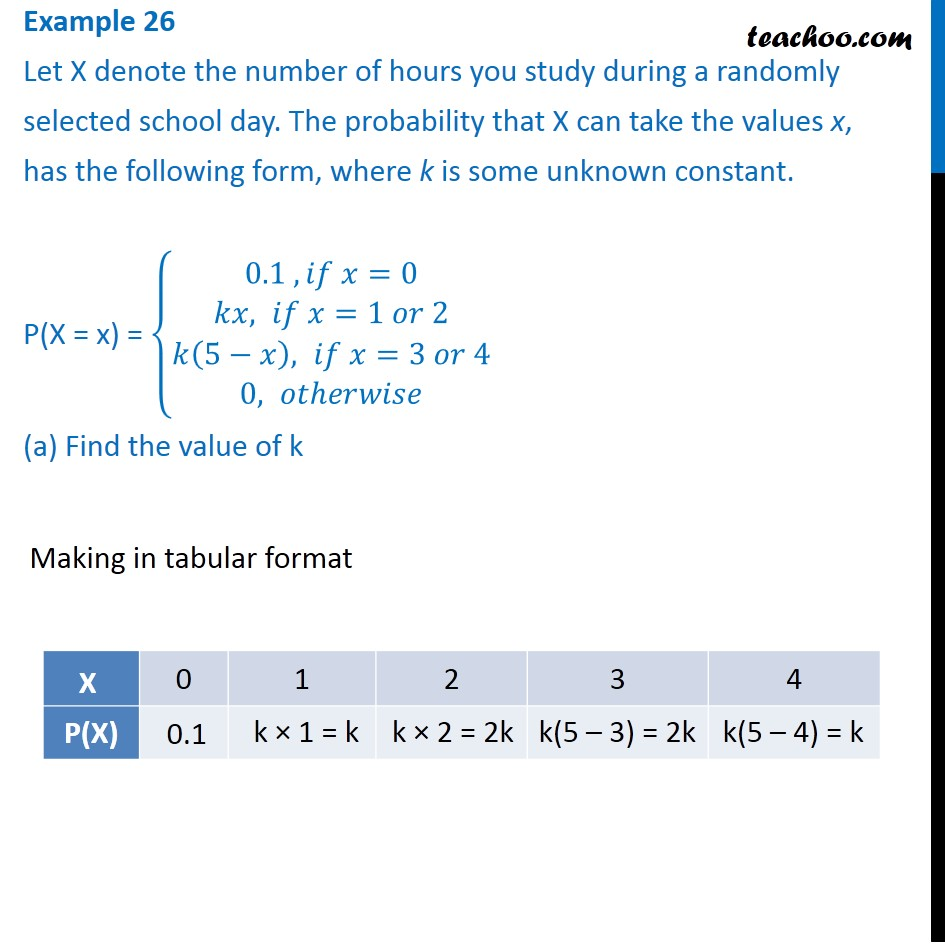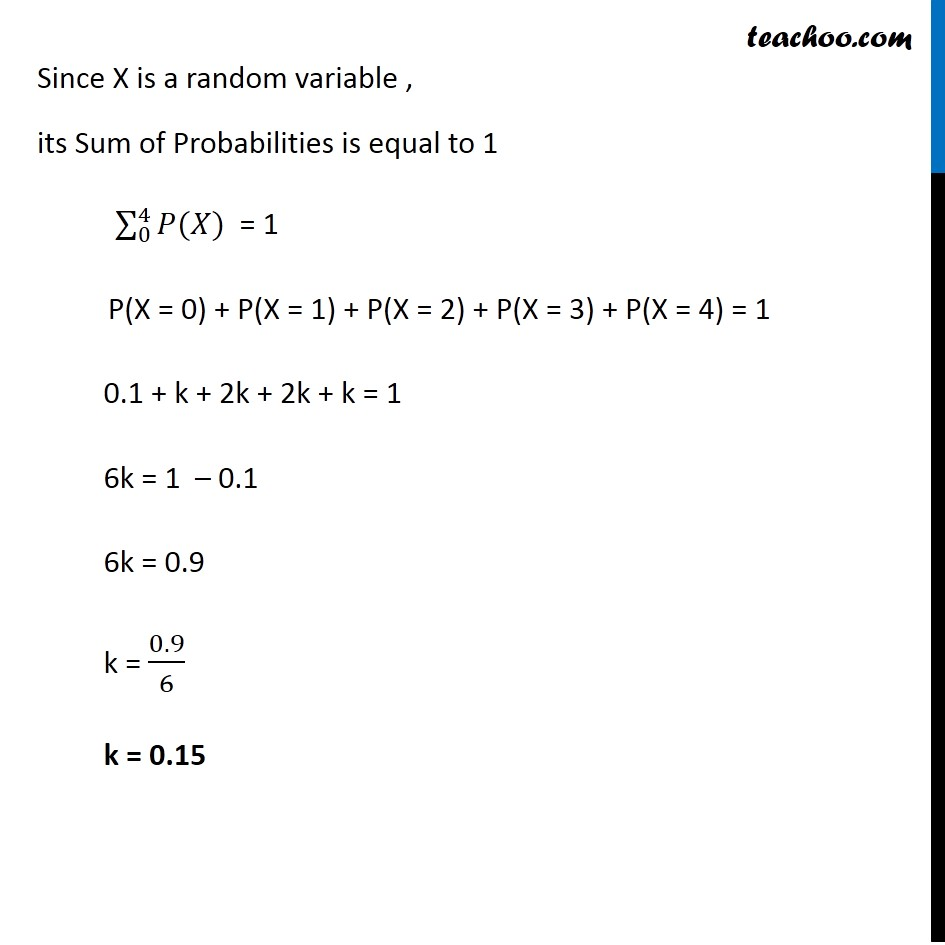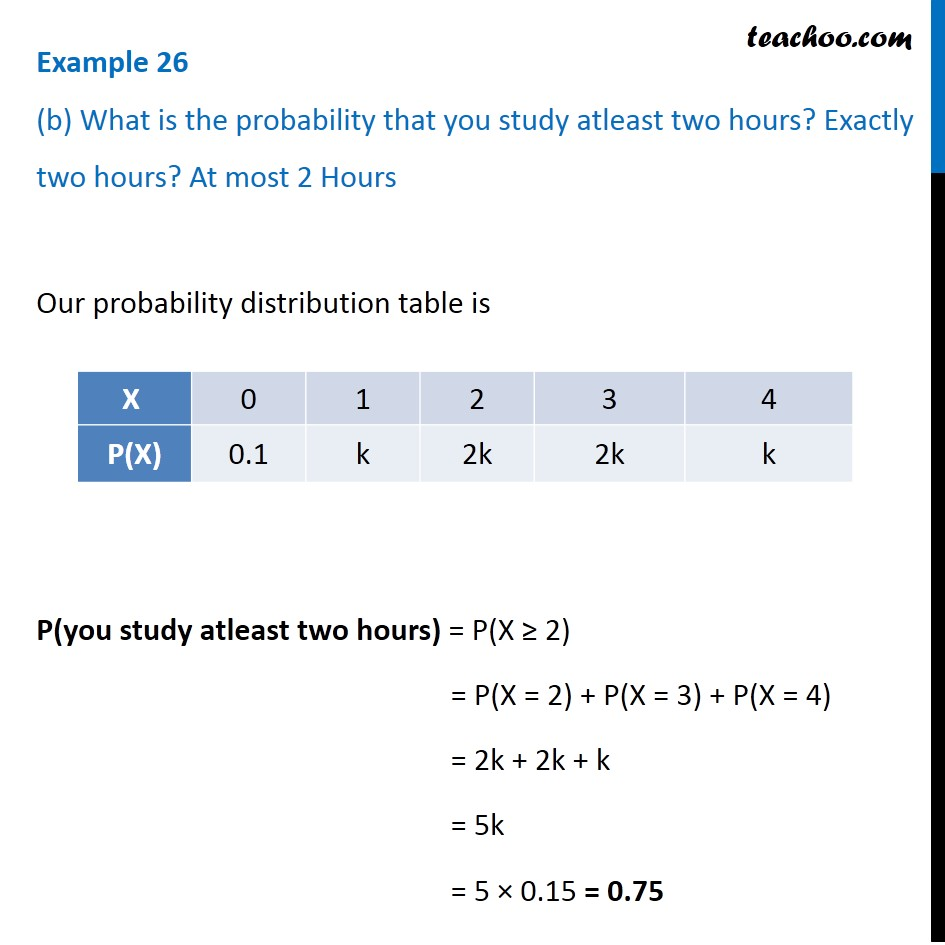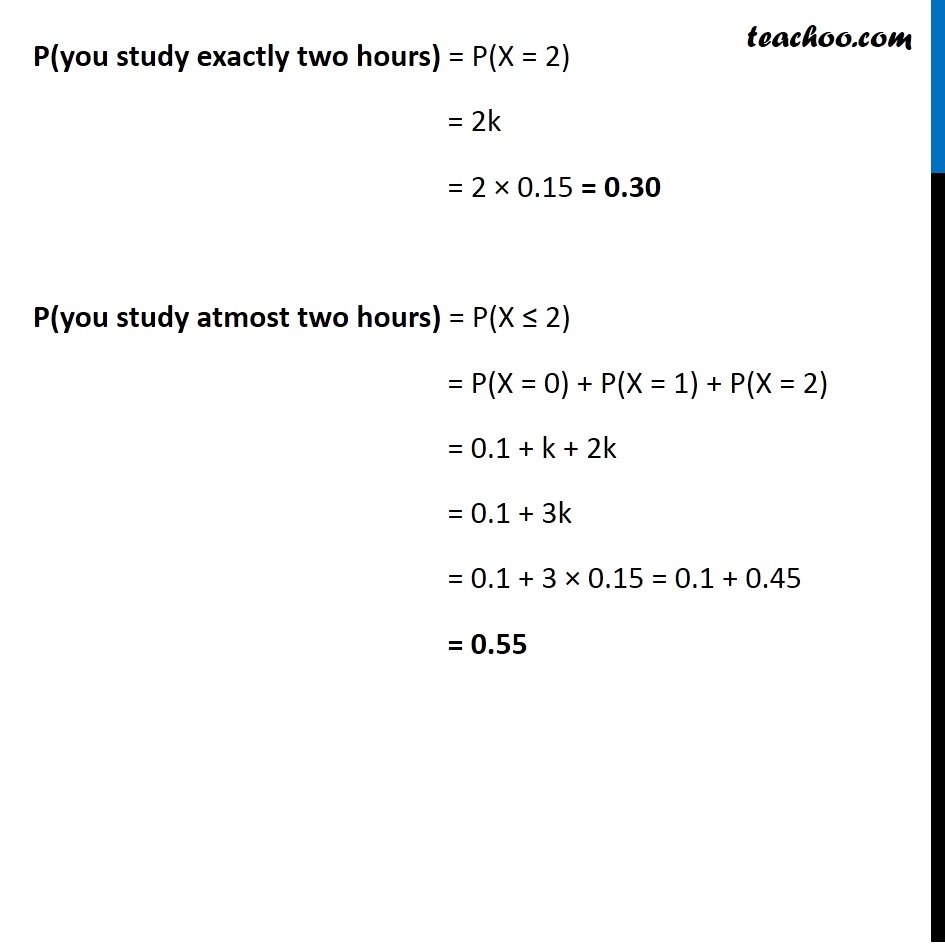Chapter 13 Class 12 Probability

Class 12
Important Questions for exams Class 12Learn in your speed, with individual attention - Teachoo Maths 1-on-1 Class

### Transcript

Question 5 Let X denote the number of hours you study during a randomly selected school day. The probability that X can take the values x, has the following form, where k is some unknown constant. P(X = x) = {█(0.1 , 𝑖𝑓 𝑥=0@𝑘𝑥, 𝑖𝑓 𝑥=1 𝑜𝑟 2@𝑘(5−𝑥), 𝑖𝑓 𝑥=3 𝑜𝑟 [email protected], 𝑜𝑡ℎ𝑒𝑟𝑤𝑖𝑠𝑒)┤ (a) Find the value of kMaking in tabular foArmat Since X is a random variable , its Sum of Probabilities is equal to 1 ∑_0^4▒〖𝑃(𝑋)〗 = 1 P(X = 0) + P(X = 1) + P(X = 2) + P(X = 3) + P(X = 4) = 1 0.1 + k + 2k + 2k + k = 1 6k = 1 – 0.1 6k = 0.9 k = (0. 9)/6 k = 0.15 Question 5 (b) What is the probability that you study atleast two hours? Exactly two hours? At most 2 HoursOur probability distribution table is P(you study atleast two hours) = P(X ≥ 2) = P(X = 2) + P(X = 3) + P(X = 4) = 2k + 2k + k = 5k = 5 × 0.15 = 0.75 P(you study exactly two hours) = P(X = 2) = 2k = 2 × 0.15 = 0.30 P(you study atmost two hours) = P(X ≤ 2) = P(X = 0) + P(X = 1) + P(X = 2) = 0.1 + k + 2k = 0.1 + 3k = 0.1 + 3 × 0.15 = 0.1 + 0.45 = 0.55